# 3.4 Case Study: N-body Simulation

In this section, we consider a new program that exemplifies object-oriented programming. Our task is to compose a program that dynamically simulates the motion of n bodies under the influence of mutual gravitational attraction. This n-body simulation problem was first formulated by Isaac Newton over 350 years ago, and scientists still study it intensely today.

One reason that this problem is a compelling example of object-oriented programming is that it presents a direct and natural correspondence between physical objects in the real world and the abstract objects that we use in programming.

## N-Body Simulation

The bouncing-ball simulation of Section 1.5 is based on Newton's first law of motion: a body in motion remains in motion at the same velocity unless acted on by an outside force. Embellishing that example to include Newton's second law of motion (which explains how outside forces affect velocity) leads us to a basic problem that has fascinated scientists for ages. Given a system of n bodies, mutually affected by gravitational forces, the problem is to describe their motion.

### Body data type.

In bouncingball.py (from Section 1.5), we keep the displacement from the origin in the floats `rx` and `ry` and the velocity in the floats `vx` and `vy`, and displace the ball the amount it moves in one time unit with the statements:

 ```rx = rx + vx ry = ry + vy ```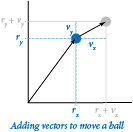With `Vector`, as defined in vector.py (from Section 3.3), we can keep the position in the Vector `r` and the velocity in the `Vector` `v`, and then displace the body the amount it moves in `dt` time units with a single statement:

 ```r = r + v.scale(dt) ```

The program body.py implements `Body`, a Python class for a data type for moving bodies. `Body` is a `Vector` client — the values of the data type are `Vector` objects that carry the body's position and velocity, as well as a float that carries the mass. The data-type operations allow clients to move and to draw the body (and to compute the force vector due to gravitational attraction from another body), as defined by this API: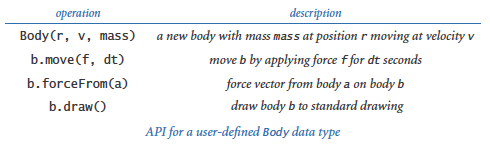Technically, the body's position (displacement from the origin) is not a vector (it is a point in space, not a direction and a magnitude), but it is convenient to represent it as a `Vector` because `Vector` operations lead to compact code for the transformation that we need to move the body. When we move a `Body`, we need to change not just its position, but also its velocity.

### Force and motion.

Newton's second law of motion says that the force on a body (a vector) is equal to the scalar product of its mass and its acceleration (also a vector): F = m a. In other words, to compute the acceleration of a body, we compute the force, then divide by its mass. In `Body`, the force is a `Vector` argument `f` to `move()`, so that we can first compute the acceleration vector just by dividing by the mass (a scalar value that is kept as a float in an instance variable) and then compute the change in velocity by adding to it the amount this vector changes over the time interval (in the same way that we used the velocity to change the position). This law immediately translates to the following code for updating the position and velocity of a body due to a given force vector `f` and amount of time `dt`:

 ```a = f.scale(1.0 / mass) v = v + a.scale(dt) r = r + v.scale(dt) ```

This code appears in the `move()` method in `Body`, to adjust its values to reflect the consequences of that force being applied for that amount of time: the body moves and its velocity changes. This calculation assumes that the acceleration is constant during the time interval.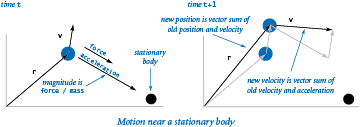### Forces among bodies.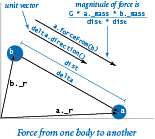The computation of the force imposed by one body on another is encapsulated in the method `forceFrom()` in `Body`, which takes a `Body` object as argument and returns a `Vector`. Newton's law of universal gravitation is the basis for the calculation: the magnitude of the gravitational force between two bodies is given by the product of their masses divided by the square of the distance between them (scaled by the gravitational constant G, which is 6.67 × 10-11 N m2 / kg2) and the direction of the force is the line between the two particles. This law translates to the following code for computing `a.forceFrom(b)`:

 ```G = 6.67e-11 delta = b._r - a._r dist = abs(delta) magnitude = G * a.mass * b.mass / (dist * dist) f = delta.direction().scale(magnitude) ```

The magnitude of the force vector is the float magnitude, and the direction of the force vector is the same as the direction of the difference vector between the two body's positions. The force vector `f` is the product of magnitude and direction.

### Universe data type.

`Universe`, as defined in universe.py, is a data type that implements the following API:Its data-type values define a universe (its size, the number of bodies, and an array of bodies) and two data-type operations: `increaseTime()`, which adjusts the positions (and velocities) of all of the bodies, and `draw()`, which draws all of the bodies. The key to the n-body simulation is the implementation of `increaseTime()` in `Universe`. The first part of the computation is a doubly nested loop that computes the force vector describing the gravitational force of each body on each other body. It applies the principle of superposition, which says that we can add together the force vectors affecting a body to get a single vector representing the aggregate force. After it has computed all of the forces, it calls the `move()` method for each body to apply the computed force for a fixed time quantum.

### File format.

The constructor reads the universe parameters and body descriptions from a file that contains the following information:

• The number of bodies
• The radius of the universe
• The position, velocity, and mass of each body

The files 2bodytiny.txt, 2body.txt, 3body.txt, and 4body.txt, contain data of that form. As usual, for consistency, all measurements are in standard SI units (recall that the gravitational constant G also appears in our code). With this defined file format, the code for our `Universe` constructor is straightforward. Each `Body` is characterized by five floats: the x- and y-coordinates of its position, the x- and y-components of its initial velocity, and its mass.

The static images shown below were made by modifying `Universe` and `Body` to draw the bodies in white, and then black on a gray background. By comparison, the dynamic images that you get when you run universe.py as it stands give a realistic feeling of the bodies orbiting one another, which is difficult to discern in the fixed pictures.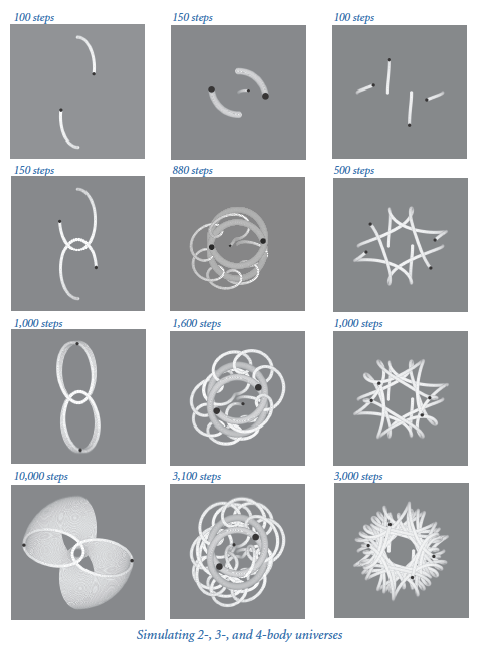#### Q & A

Q. The `Universe` API is certainly small. Why not just implement that code in a main() test client for `Body`?

A. Our design is an expression of what many people believe about the universe: it was created, and then time moves on. It clarifies the code and allows for maximum flexibility in simulating what goes on in the universe.

Q. Why is `forceFrom()` a method? Wouldn't it be better for it to be a function that takes two `Body` objects as arguments?

A. Implementing `forceFrom()` as a method is one of several possible alternatives, and having a function that takes two `Body` objects as arguments is certainly a reasonable choice. Some programmers prefer to completely avoid functions in data-type implementations; another option is to maintain the force acting on each `Body` as an instance variable. Our choice is a compromise between these two options.

Q. Shouldn't the `move()` method in body.py update the position using the old velocity instead of the updated velocity?

A. It turns out that using the updated velocity (known as the leapfrog method) produces more accurate results than using the old velocity (known as the Euler method). If you take a course in numerical analysis, you will learn why.

#### Exercises

1. Develop an object-oriented version of bouncingball.py (from Section 1.5). Include a constructor that starts each ball moving a random direction at a random velocity (within reasonable limits) and a test client that takes an integer `n` from the command line and simulates the motion of `n` bouncing balls.

2. Add to body.py a `main()` function that unit tests the Body data type.

3. Modify body.py so that the radius of the circle it draws for a body is proportional to its mass.

4. What happens in a universe with no gravitational force? This situation would correspond to `forceFrom()` in `Body` always returning the zero vector.

5. Create a data type `Universe3D` to model three-dimensional universes. Develop a data file to simulate the motion of the planets in our solar system around the sun.

6. Compose a test client that simulates the motion of two different universes (defined by two different files and appearing in two distinct parts of the standard drawing window). You also need to modify the `draw()` method in `Body`.

7. Compose a class `RandomBody` that initializes its instance variables with (carefully chosen) random values instead of taking them as arguments. Then compose a client that takes a single argument `n` from the command line and simulates motion in a random universe with `n` bodies.

8. Modify `Vector` (as defined in vector.py from Section 3.3) to include a method `__iadd__(self, other)` to support the in-place addition operator `+=`, which enables the client to compose code like `r += v.scale(dt)`. Using this method, revise body.py and universe.py.

9. Modify the `Vector` constructor (as defined in vector.py from Section 3.3) so that if passed a positive integer `d` as argument, it creates and returns the all-zero vector of dimension `d`. Using this modified constructor, revise universe.py so that it works with three- (or higher-) dimensional universes. For simplicity, don't worry about changing the `draw()` method in body.py — it projects the position onto the plane defined by the first x- and y-coordinates.

#### Creative Exercise

1. New universe. Design a new universe with interesting properties and simulate its motion with `Universe`, as defined in universe.py. This exercise is truly an opportunity to be creative!

2. Percolation. Compose an object-oriented version of percolation.py (from Section 2.4). Think carefully about the design before you begin, and be prepared to defend your design decisions.